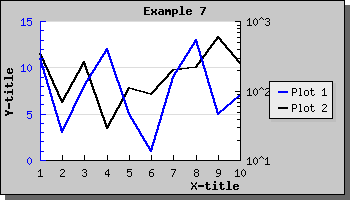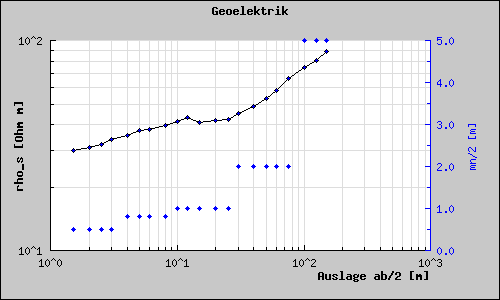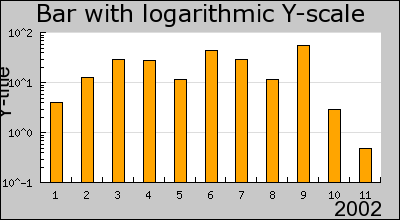Contents Previous Next

### 8.1.8 Using logarithmic scale

Using a logarithmic scale requires you to include the logarithmic add on module in "jpgraph_log.php". So you must have the line

```   include("jpgraph_log.php" );```

on the top of your code. To Illustrate how to use a logarithmic scale let's make the right Y scale in the previous example a logarithmic scale. This is done by the line

```  \$graph->SetY2Scale( "log"); ```

This will then give the following resultFigure 15: Using a logarithmic scale for both the Y2 axis [src]

You can of course also use a logarithmic X-scale as well. The following example shows this.Figure 16: Example of using log scale on both X and Y axis together with a linear Y2 scale [src]

Even though we have so far only shown line graphs logarithmic scale can also be used for bar, error, scatter plots as well. Even radar plots supports the use of logarithmic plots. The following example shows how to use a logarithmic scale for a bar graph.Figure 17: Example of using logarithmic scale togther with bar plots [src]

Contents Previous Next